• No products in the cart.

# 204.5.7 The Neural Network Algorithm

##### Steps of neural network algorithm.

Link to the previous post : https://statinfer.com/204-5-6-neural-network-intuition/

In all the previous posts we progressed to this part. We will breakdown the steps how a neural network starts and ends.

### The Neural Network Algorithm

• Step 1: Initialization of weights: Randomly select some weights.
• Step 2 : Training & Activation: Input the training values and perform the calculations forward.
• Step 3 : Error Calculation: Calculate the error at the outputs. Use the output error to calculate error fractions at each hidden layer.
• Step 4: Weight training: Update the weights to reduce the error, recalculate and repeat the process of training & updating the weights for all the examples.
• Step 5: Stopping criteria: Stop the training and weights updating process when the minimum error criteria is met.

Randomly Initialize Weights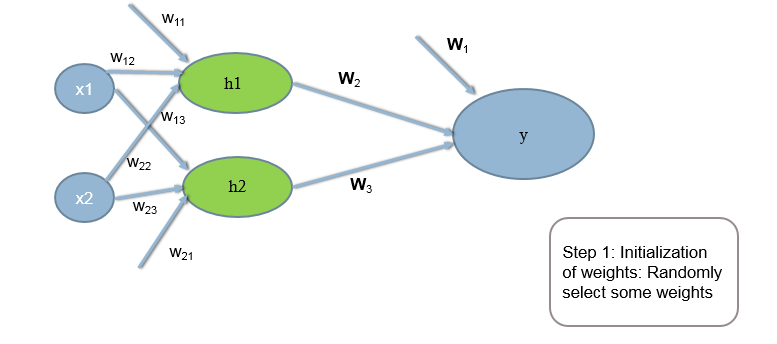Training & Activation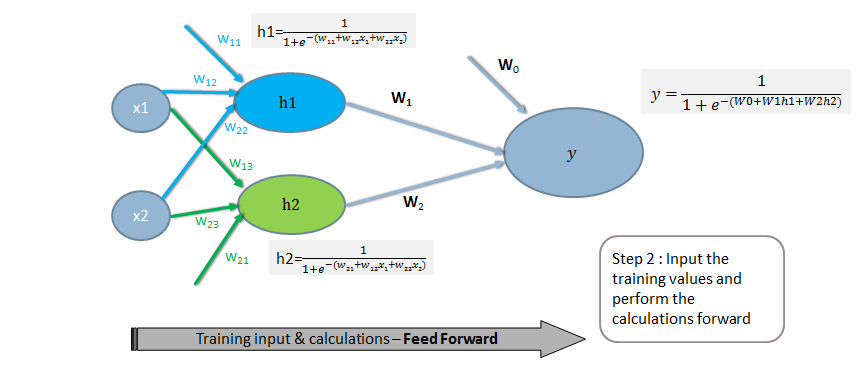Error Calculation at Output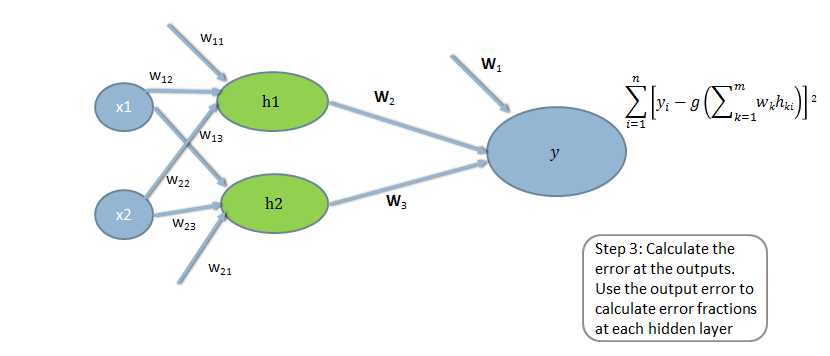Error Calculation at hidden layers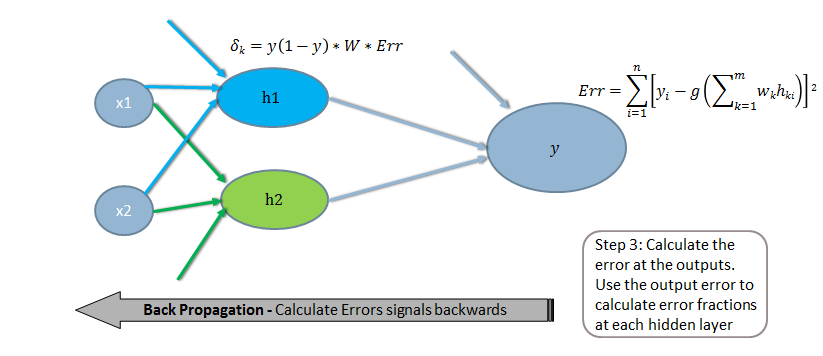Calculate weight corrections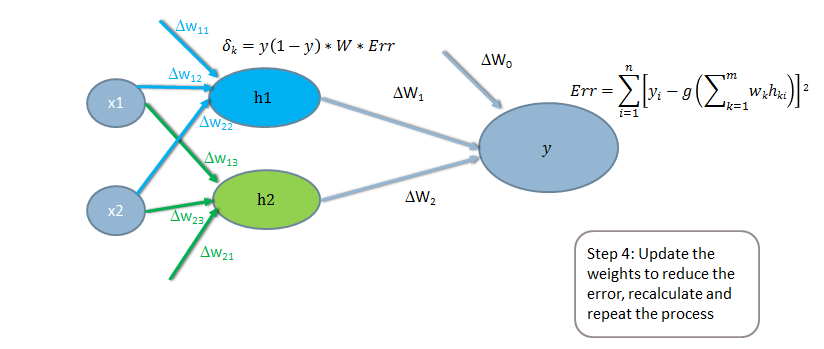Update Weights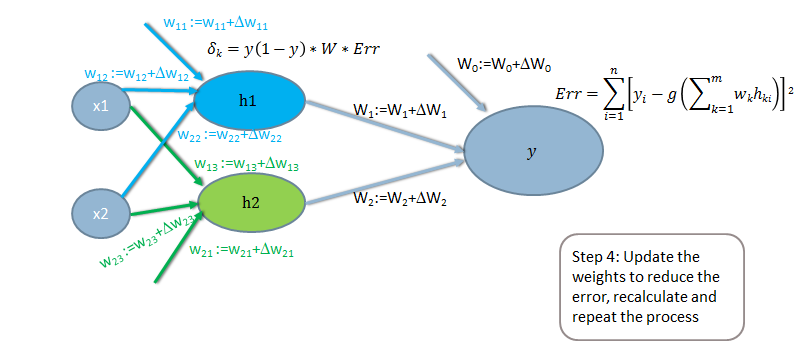Stopping Criteria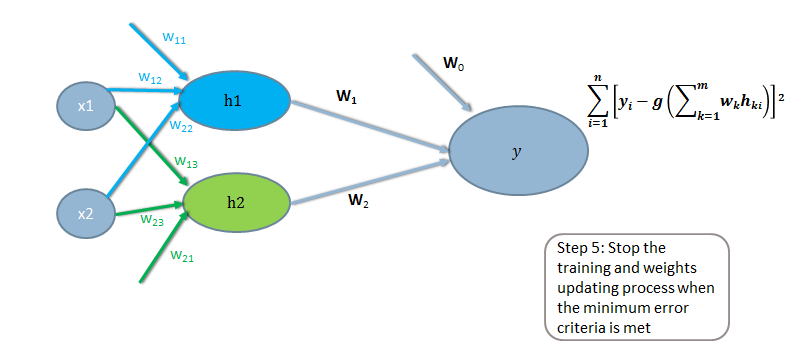```The next post is a demo on neural network algorithm.
Link to the next post : https://statinfer.com/204-5-8-neural-network-algorithm-demo/```
21st June 2017
0 responses on "204.5.7 The Neural Network Algorithm"
© 2020. All Rights Reserved.